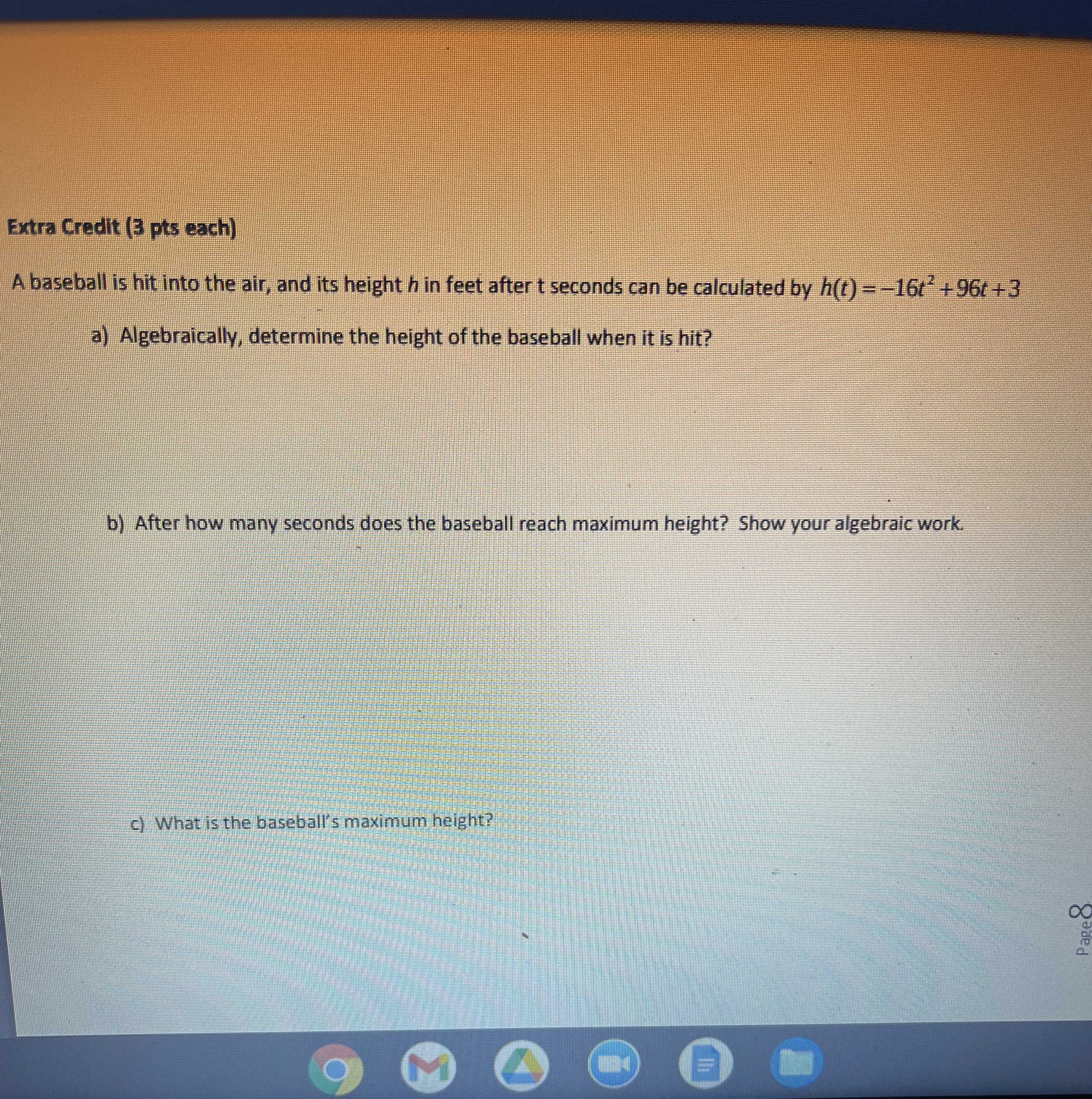### ¿Todavía tienes preguntas de matemáticas?

Pregunte a nuestros tutores expertos
Algebra
PreguntaA baseball is hit into the air, and its height $$h$$ in feet after $$t$$ seconds can be calculated by $$h ( t ) = - 16 t ^ { 2 } + 96 t + 3$$

a) Algebraically, determine the height of the baseball when it is hit?

b) After how many seconds does the baseball reach maximum height? Show your algebraic work. c) c)What is the baseball's maximum height?